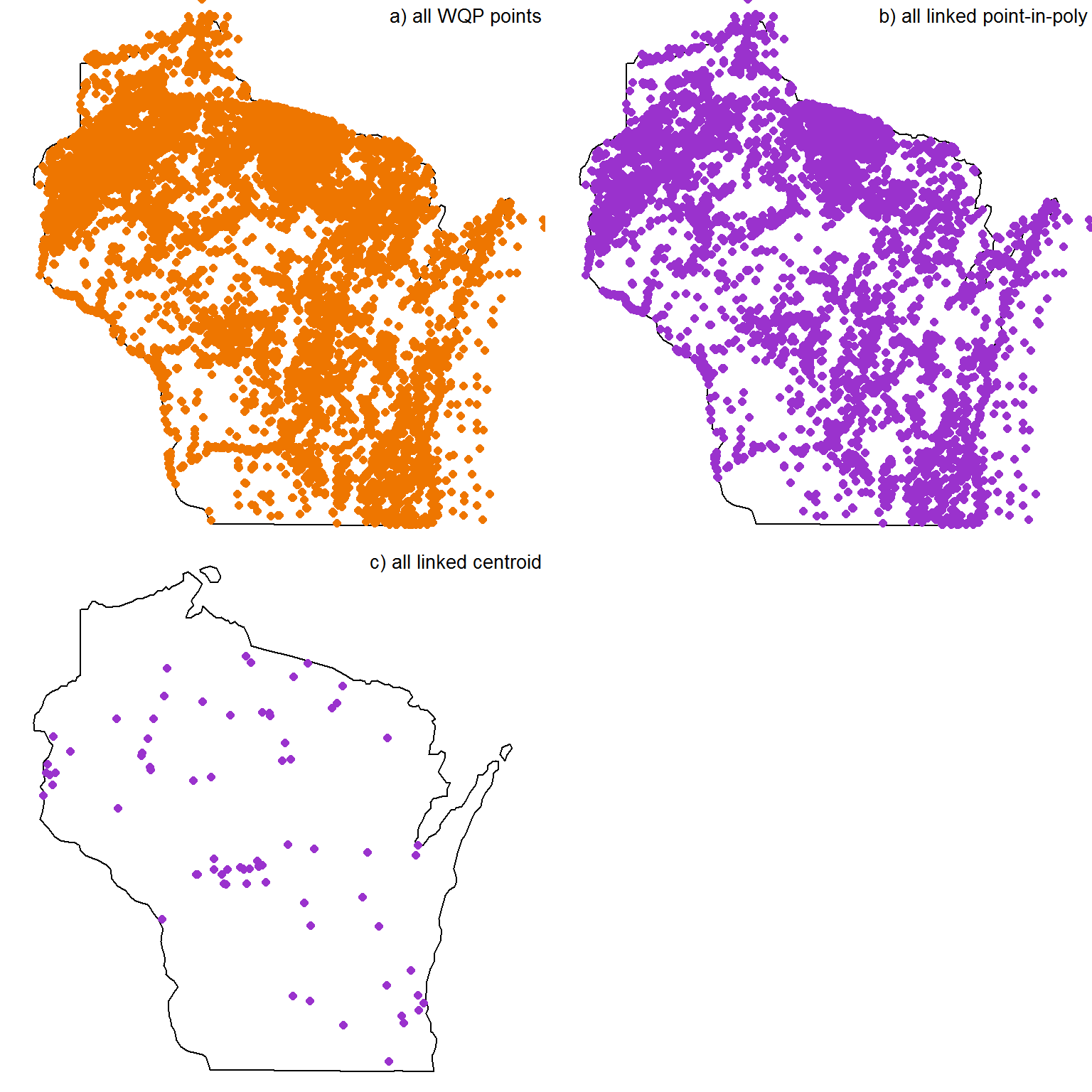This is an example of using hydrolinks to link a large, unlinked aquatic dataset to the U.S. Geological Survey’s National Hydrography Network. The unlinked aquatic dataset is from the Water Quality Portal (WQP). In this example, I will link a lakes, but you could also link streams.

The WQP data is a perfect use-case here. The data are all described by sites that have Latitude/Longitude geopoint data, but no other concrete way to link the data to the hydrologic network. So in this example, we will link all lake observation sites in the WQP to the National Hydrography Dataset high-res (NHDH). Any sites that don’t link using the simple point-in-polygon routine

First, load packages and grab lake data. I’m limiting the data to be from the state of Wisconsin initially. This just speeds up generation of the Vignette. The entire US dataset takes a bit longer than I’d like to wait to link and visualize.

library(dataRetrieval)
library(USAboundaries)
#library(ggplot2)
#library(gridExtra)
library(sf)

lsites = whatWQPsites(siteType ="Lake, Reservoir, Impoundment", statecode='US:55')

At the point of this Vignette generation, there were 19356 unique sites returned by this query.

Now, lets link all these sites to the NHDH. After the initial point-in-polygon linking, I will take the remainder and use the centroid matching.

linked_lakes = link_to_waterbodies(lsites$LatitudeMeasure, lsites$LongitudeMeasure, lsites$MonitoringLocationIdentifier, dataset = 'nhdh', buffer = 0) unlinked = subset(lsites, !(MonitoringLocationIdentifier %in% linked_lakes$MATCH_ID))
linked_lcent = link_waterbody_centroids(unlinked$LatitudeMeasure, unlinked$LongitudeMeasure, unlinked$MonitoringLocationIdentifier, dataset = 'nhdh', buffer = 25) Here are the overarching number of sites linked/centroid-linked/not-linked. Link Count Point-in-polygon 10002 Centroid 77 Unlinked 9278 Total 19356 Lastly, lets visualize these sites. Just mash them all together. (also left in bleeding edge geom_sf code that fails on CRAN). wi = USAboundaries::us_boundaries()[USAboundaries::us_boundaries()$geoid %in% c('55'),]
all_points = st_as_sf(lsites, coords = c("LongitudeMeasure", "LatitudeMeasure"), crs = '+proj=longlat +ellps=WGS84 +datum=WGS84 +no_defs')

par(mfrow=c(2,2), mar=c(0,0,0,0))
plot(st_geometry(wi))
legend('topright', legend=' ', title='a) all WQP points', bty='n')

pippoints = subset(all_points, MonitoringLocationIdentifier %in% linked_lakes$MATCH_ID) plot(st_geometry(wi)) plot(st_geometry(pippoints), col='darkorchid3', add=TRUE, pch=16) legend('topright', legend=' ', title='b) all linked point-in-poly', bty='n') centpoints = subset(all_points, MonitoringLocationIdentifier %in% linked_lcent$MATCH_ID)
plot(st_geometry(wi))
legend('topright', legend=' ', title='c) all linked centroid', bty='n')

# gall = ggplot(all_points) +
#   geom_sf(data=wi, fill='grey') +
#   geom_sf(size=0.5, color='darkorange2') + ylim(42, 47) + xlim(-93, -86.5) +
#   ggtitle('a) all WQP points')
#
# glink = ggplot(subset(all_points, MonitoringLocationIdentifier %in% linked_lakes$MATCH_ID)) + # geom_sf(data=wi, fill='grey') + # geom_sf(size=0.5, color='dodgerblue') + ylim(42, 47) + xlim(-93, -86.5) + # ggtitle('b) all linked point-in-poly') # # gcent = ggplot(subset(all_points, MonitoringLocationIdentifier %in% linked_lcent$MATCH_ID)) +
#   geom_sf(data=wi, fill='grey') +
#   geom_sf(size=0.5, color='darkorchid3') + ylim(42, 47) + xlim(-93, -86.5) +
# grid.arrange(gall, glink, gcent, nrow = 2)